Search by Topic

Resources tagged with Interactivities similar to Connect Three:

Filter by: Content type:
Age range:
Challenge level:

There are 139 results

Broad Topics > Information and Communications Technology > InteractivitiesConnect Three

Age 11 to 16 Challenge Level:

Can you be the first to complete a row of three?First Connect Three

Age 7 to 14 Challenge Level:

The idea of this game is to add or subtract the two numbers on the dice and cover the result on the grid, trying to get a line of three. Are there some numbers that are good to aim for?Excel Interactive Resource: Haitch

Age 11 to 16 Challenge Level:

An Excel spreadsheet with an investigation.Game of PIG - Sixes

Age 7 to 18 Challenge Level:

Can you beat Piggy in this simple dice game? Can you figure out Piggy's strategy, and is there a better one?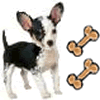Ratio Pairs 3

Age 11 to 16 Challenge Level:

Match pairs of cards so that they have equivalent ratios.White Box

Age 7 to 18 Challenge Level:

This game challenges you to locate hidden triangles in The White Box by firing rays and observing where the rays exit the Box.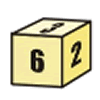First Connect Three for Two

Age 7 to 14 Challenge Level:

First Connect Three game for an adult and child. Use the dice numbers and either addition or subtraction to get three numbers in a straight line.Excel Interactive Resource: Long Multiplication

Age 11 to 16 Challenge Level:

Use an Excel spreadsheet to explore long multiplication.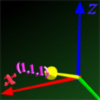Tracking Points

Age 14 to 16 Challenge Level:

Can you give the coordinates of the vertices of the fifth point in the patterm on this 3D grid?Spinners Environment

Age 5 to 18 Challenge Level:

A tool for generating random integers.Countdown Fractions

Age 11 to 16 Challenge Level:

Here is a chance to play a fractions version of the classic Countdown Game.Inside Out

Age 14 to 16 Challenge Level:

There are 27 small cubes in a 3 x 3 x 3 cube, 54 faces being visible at any one time. Is it possible to reorganise these cubes so that by dipping the large cube into a pot of paint three times you. . . .Cubic Net

Age 14 to 18 Challenge Level:

This is an interactive net of a Rubik's cube. Twists of the 3D cube become mixes of the squares on the 2D net. Have a play and see how many scrambles you can undo!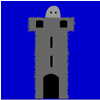Tower Rescue

Age 7 to 18 Challenge Level:

Help the bee to build a stack of blocks far enough to save his friend trapped in the tower.Squaring the Rectangle

Age 14 to 18 Challenge Level:

Can you find a way to turn a rectangle into a square?Konigsberg Plus

Age 11 to 14 Challenge Level:

Euler discussed whether or not it was possible to stroll around Koenigsberg crossing each of its seven bridges exactly once. Experiment with different numbers of islands and bridges.Sliding Puzzle

Age 5 to 16 Challenge Level:

The aim of the game is to slide the green square from the top right hand corner to the bottom left hand corner in the least number of moves.Jam

Age 14 to 16 Challenge Level:

To avoid losing think of another very well known game where the patterns of play are similar.Excel Interactive Resource: Fraction Multiplication

Age 11 to 16 Challenge Level:

Use Excel to explore multiplication of fractions.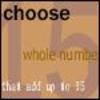Fifteen

Age 7 to 14 Challenge Level:

Can you spot the similarities between this game and other games you know? The aim is to choose 3 numbers that total 15.Online

Age 7 to 14 Challenge Level:

A game for 2 players that can be played online. Players take it in turns to select a word from the 9 words given. The aim is to select all the occurrences of the same letter.Got it Article

Age 7 to 14

This article gives you a few ideas for understanding the Got It! game and how you might find a winning strategy.Nim-interactive

Age 11 to 16 Challenge Level:

Start with any number of counters in any number of piles. 2 players take it in turns to remove any number of counters from a single pile. The winner is the player to take the last counter.Cushion Ball Interactivity

Age 14 to 16 Challenge Level:

The interactive diagram has two labelled points, A and B. It is designed to be used with the problem "Cushion Ball"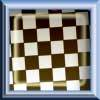Diagonal Dodge

Age 7 to 14 Challenge Level:

A game for 2 players. Can be played online. One player has 1 red counter, the other has 4 blue. The red counter needs to reach the other side, and the blue needs to trap the red.Lost on Alpha Prime

Age 14 to 16 Challenge Level:

On the 3D grid a strange (and deadly) animal is lurking. Using the tracking system can you locate this creature as quickly as possible?Excel Interactive Resource: Interactive Division

Age 11 to 16 Challenge Level:

Use an Excel to investigate division. Explore the relationships between the process elements using an interactive spreadsheet.Instant Insanity

Age 11 to 18 Challenge Level:

Given the nets of 4 cubes with the faces coloured in 4 colours, build a tower so that on each vertical wall no colour is repeated, that is all 4 colours appear.Changing Places

Age 14 to 16 Challenge Level:

Place a red counter in the top left corner of a 4x4 array, which is covered by 14 other smaller counters, leaving a gap in the bottom right hand corner (HOME). What is the smallest number of moves. . . .Factors and Multiples - Secondary Resources

Age 11 to 16 Challenge Level:

A collection of resources to support work on Factors and Multiples at Secondary level.Percentages for Key Stage 4

Age 14 to 16 Challenge Level:

A group of interactive resources to support work on percentages Key Stage 4.Excel Interactive Resource: Fraction Addition & Fraction Subtraction

Age 11 to 16 Challenge Level:

Use Excel to practise adding and subtracting fractions.Just Rolling Round

Age 14 to 16 Challenge Level:

P is a point on the circumference of a circle radius r which rolls, without slipping, inside a circle of radius 2r. What is the locus of P?Loci Resources

Age 14 to 16 Challenge Level:

This set of resources for teachers offers interactive environments to support work on loci at Key Stage 4.Fractions and Percentages Card Game

Age 11 to 16 Challenge Level:

Match the cards of the same value.Got a Strategy for Last Biscuit?

Age 11 to 16 Challenge Level:

Can you beat the computer in the challenging strategy game?Square It

Age 11 to 16 Challenge Level:

Players take it in turns to choose a dot on the grid. The winner is the first to have four dots that can be joined to form a square.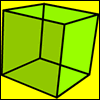Cubic Rotations

Age 14 to 16 Challenge Level:

There are thirteen axes of rotational symmetry of a unit cube. Describe them all. What is the average length of the parts of the axes of symmetry which lie inside the cube?Solving with Euclid's Algorithm

Age 14 to 18 Challenge Level:

A java applet that takes you through the steps needed to solve a Diophantine equation of the form Px+Qy=1 using Euclid's algorithm.Number Pyramids

Age 11 to 14 Challenge Level:

Try entering different sets of numbers in the number pyramids. How does the total at the top change?Flip Flop - Matching Cards

Age 5 to 14 Challenge Level:

A game for 1 person to play on screen. Practise your number bonds whilst improving your memoryExcel Interactive Resource: the up and Down Game

Age 11 to 16 Challenge Level:

Use an interactive Excel spreadsheet to explore number in this exciting game!Excel Interactive Resource: Number Grid Functions

Age 11 to 16 Challenge Level:

Use Excel to investigate the effect of translations around a number grid.Excel Interactive Resource: Multiples Chain

Age 11 to 16 Challenge Level:

Use an interactive Excel spreadsheet to investigate factors and multiples.Excel Interactive Resource: Equivalent Fraction Bars

Age 11 to 16 Challenge Level:

A simple file for the Interactive whiteboard or PC screen, demonstrating equivalent fractions.Icosian Game

Age 11 to 14 Challenge Level:

This problem is about investigating whether it is possible to start at one vertex of a platonic solid and visit every other vertex once only returning to the vertex you started at.Disappearing Square

Age 11 to 14 Challenge Level:

Do you know how to find the area of a triangle? You can count the squares. What happens if we turn the triangle on end? Press the button and see. Try counting the number of units in the triangle now. . . .Countdown

Age 7 to 14 Challenge Level:

Here is a chance to play a version of the classic Countdown Game.Drips

Age 7 to 14 Challenge Level:

An animation that helps you understand the game of Nim.